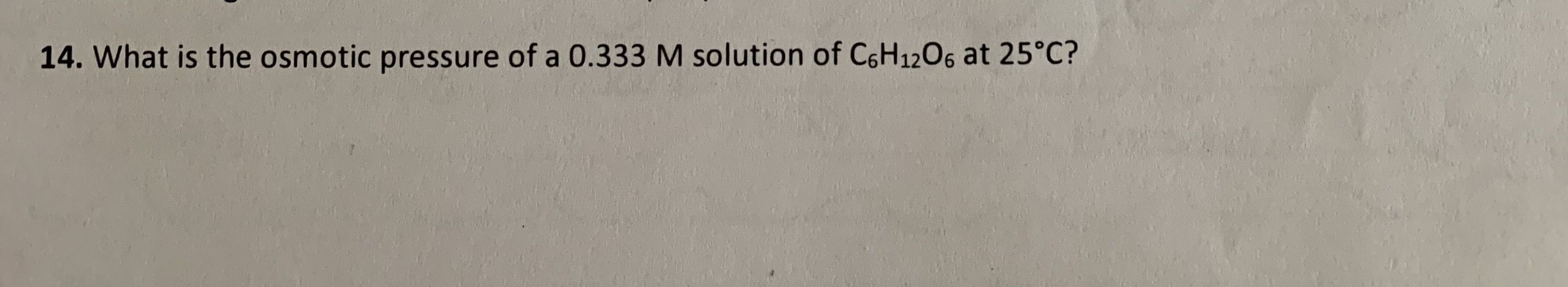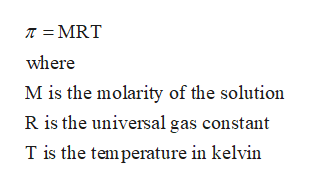# 14. What is the osmotic pressure of a 0.333 M solution of C6H1206 at 25°C?

Question
1 viewshelp_outlineImage Transcriptionclose14. What is the osmotic pressure of a 0.333 M solution of C6H1206 at 25°C? fullscreen
check_circle

Step 1

Given

Molarity of the given glucose solution = 0.333M

Temperature = 25°C

= (25+273)K =298K

Step 2

Osmotic pressure is denoted by π and is given byhelp_outlineImage TranscriptioncloseT = MRT where M is the molarity of the solution R is the universal gas constant T is the temperature in kelvin fullscreen
Step 3

The osmotic pressure of t...

### Want to see the full answer?

See Solution

#### Want to see this answer and more?

Solutions are written by subject experts who are available 24/7. Questions are typically answered within 1 hour.*

See Solution
*Response times may vary by subject and question.
Tagged in

### Chemistry